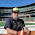### Proof of -1 * -1 = 1

Prove that minus one times minus one equals one. It's such a simple statement that many people wouldn't know where to begin. The hitch is that you're only given the 9 axioms of algebra. My father worked on this problem for a few hours for his graduate math class this semester at SJSU before he gave up and let me take a shot at it. I feel that I just got lucky and saw the solution after playing around with it for 15 minutes.

A1) a + b = b + a
A2) (a + b) + c = a + (b + c)
A3) 0 + a = a and a + 0 = a
A4) a + (-a) = 0 and (-a) + a = 0
M1) a * b = b * a
M2) (a * b) * c = a * (b * c)
M3) 1 * a = a and a * 1 = a
M4) a * (1/a) = 1 and (1/a) * a = 1
D) a * (b + c) = (a * b) + (a * c)

And on top of those, knowing that 0 * a = 0 would make our lives a little easier, so let us prove that theorem:
Start) a = a
M3) a = a * 1
A3) a = a * (1+0)
D) a = a * 1 + a * 0
M3) a = a + (a * 0) = a + 0
A3) a * 0 = 0
QED:
T1) a * 0 = 0

And now, to prove -1 * -1 = 1:
Start) -1 * -1 = 1
A3) -1 * -1 + 0 = 1
A4) -1 * -1 + -1 + 1 = 1
M3) -1 * -1 + -1 * 1 + 1 = 1
D) -1 (-1 + 1) + 1 = 1
A4) -1 (0) + 1 = 1
T1) 0 + 1 = 1
A3) 1 = 1
QED! It's proven!

And there it is. I bet you got pretty worried I wouldn't make it there towards the end, huh?

1.heh. first i thought it was gonna be the cliche 2 = 1 proof.

this is one of those where people are like "what? why would you need to =prove= that?" but at least it's concrete enough that given those axioms you have a general idea of where to start trying. As opposed to something that seems completely obvious but you wouldn't have much idea where to start. Like proving that the square root of 2 is irrational or something like that.

--DDRKirby(ISQ)

2.I'm not sure I understand line 5 of the proof of T1. Your justification is (A3), which says 0 + a = a. Where is the zero you are canceling from both sides of the step above?

73

3.thirdspectator: I'm using A3 to show that since a + 0 = a, in the expression a + (something) = a, that something must be equal to 0.

You're right though, I'm probably missing a step, or could have better articulated it. Having never taken an analysis class, it's still reflex to move stuff around without thinking about it. I'll look into it.